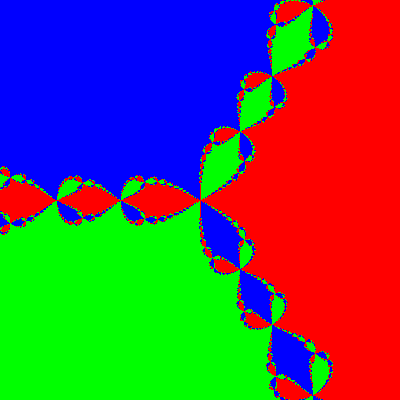The basin of attraction of a particular solution to an equation, such as a cubic polynomial, under a solution method, such as Newton's Method, is the collection of starting points that end at that solution.

For the cubic equation in the complex plane the basins of attraction are fractals.

Here we have coloured each point according to the root that Newton's Method converges to. When close to a root, that's where you end up. However, when nearly equidistant from two roots the derivative is close to zero, so the tangent can take us a long way away. Then we are starting the process from an effectively random point.Basins of attraction of the cubic $x^3=1$

(none) (none) (none)
(none) (none)

## You are here

BasinOfAttraction
ComplexPlane
CubicPolynomial
NewtonsMethod
Root
(none) (none) ArgandDiagram
ComplexNumber
CubeRoot
Euclid
Function
FundamentalTheoremOfAlgebra
ImaginaryNumber
IsaacNewton
NewtonRaphsonMethod
Polynomial
RealNumber
RiemannHypothesis
SquareRoot